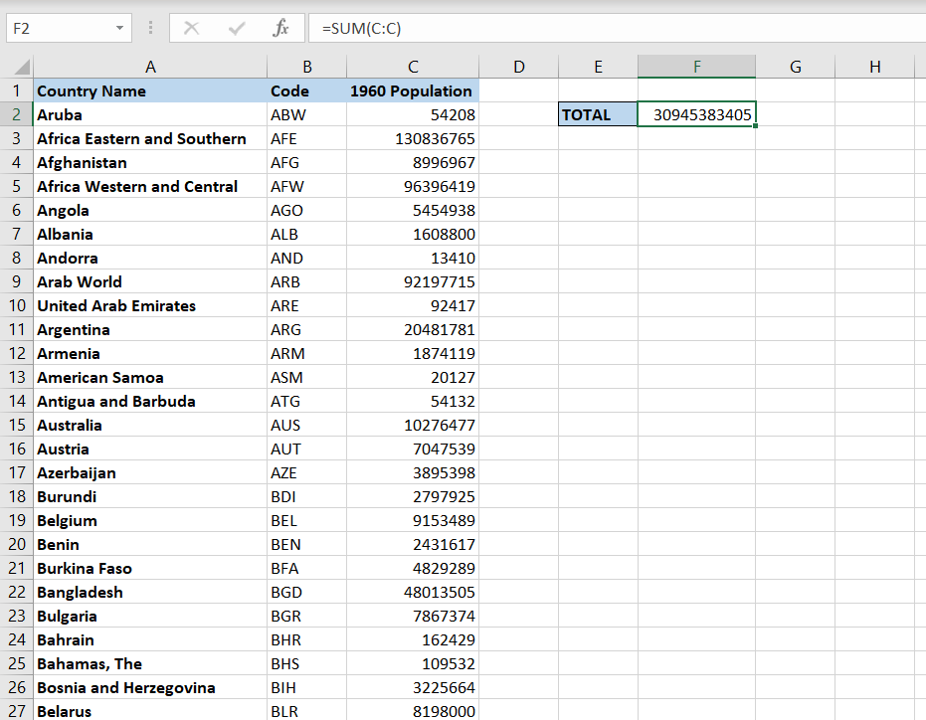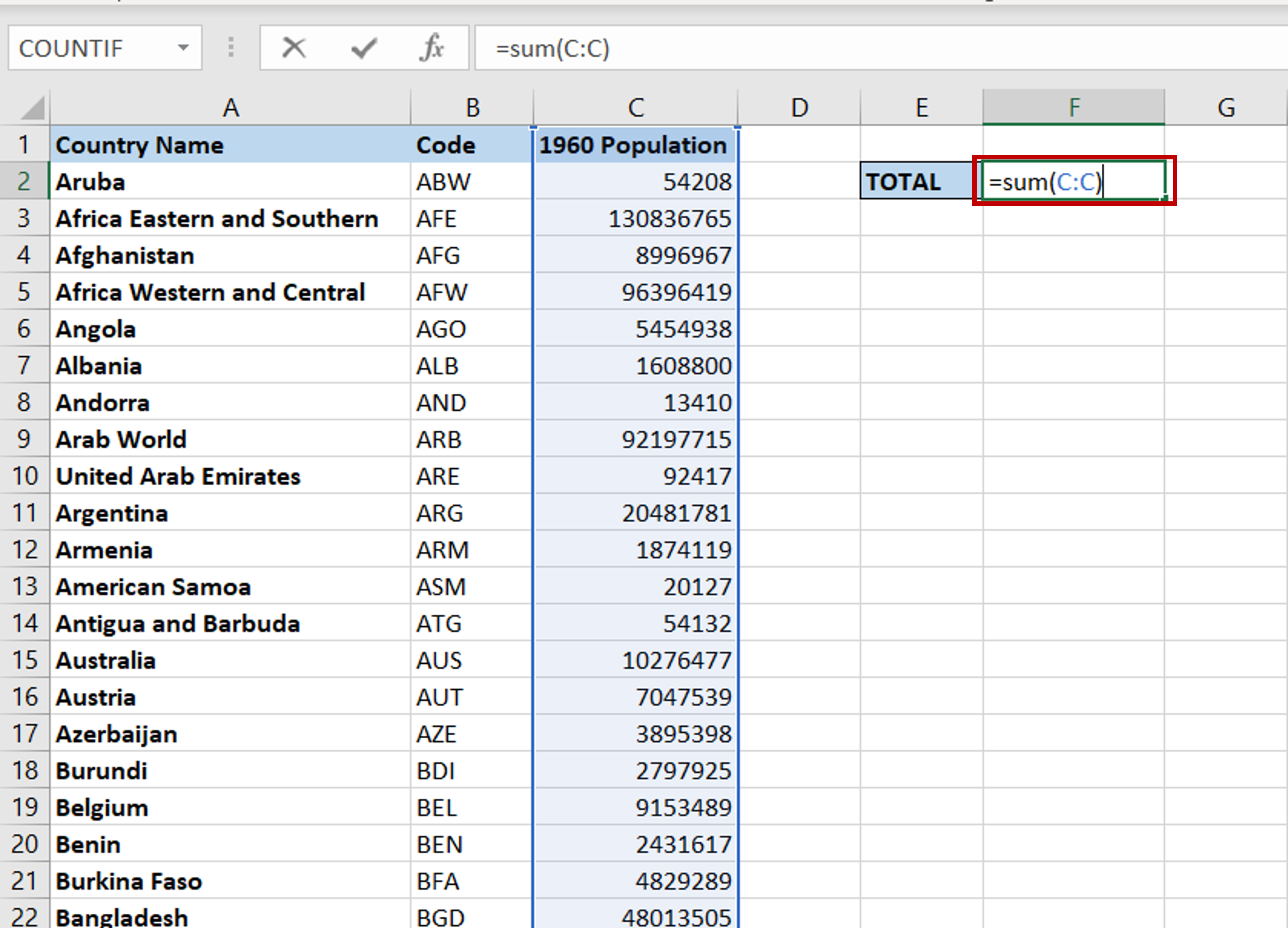# How to sum an entire column in Excel

You can watch a video tutorial here.

Excel is widely used for calculations due to the several arithmetic operators and functions that it has. When working with tables of data, you will frequently need to find the totals of columns of numbers.  If the dataset is small, it is easy to use the exact cell references when creating the formula. If the dataset is very large, it may be easier to sum the entire column.To sum a column, the SUM() function is used.

1. SUM() function: this returns the sum of a range of numbers or individual numbers
a. Syntax: SUM(numbers)
i. numbers: this is a range of numbers or individual ranges/numbers separated by a comma

### Step 1 – Create the formula– Select the cell where the result is to appear
– Type the formula:
= SUM(C:C)
– Using the column letter as the start and end of the range indicates that the entire column is to be summed
– Press Enter

### Step 2 – Check the result– All the numbers in the entire column are summed